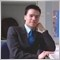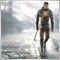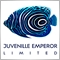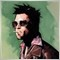• 概述
• 评论 (4)
• 评论 (11)

# Torsion GBPUSD

The Torsion GBPUSD software implements a semi-automatic trading option on GBPUSD. It runs on the H1 timeframe. The program facilitates the trading process, but does not work on the principle 'once launched and forgotten, profiting for years'. The program monitors the situation for a successful entry into the market; when a signal is received, opens a buy or a sell order depending on the signal type.

Once an order is opened, the program maintains it. If the trade is going well, then after a while a certain level of unfixed profit will be reached. Once the minimum allowed profit value is reached, the EA sets an order closing level to protect this profit in casr the "market goes the wrong way". Reaching a certain profit level, it sets the order closing level. Once these values ​​are set, the order is no longer controlled by the trading robot. The trader gets an open order with unfixed profit and then manages it himself. If you don't want to keep track of the order (or there is no possibility to do that), then the order will be closed after a while - after the maximum or minimum value of profit is reached. Once an order with unfixed profit is transferred to the trader, the trading robot waits for the next signal to enter the market.

Of course, it is possible that after the order is opened the situation changes and, after a while, you can make a prediction that the order cannot reach the desired level to be transferred to the trader. If the order is in breakeven, it will be closed. If it has unfixed loss, the EA tries to open one or more orders that will bring all the orders to a common breakeven level. After that, all open orders monitored by the program are closed.

### Operation algorithm description

1. If there are no open orders and a signal appeared (the program determines the buy or sell signal on the basis of the indicator MAGISTR AIV indicator), a buy or a sell order with the starting lot Lot is opened.
2. If only one order is open and profit of p_prib points is reached (e.g. 100 points), the order is moved to breakeven. p_min – minimum value oef fixed profit, p_max – maximum value of fixed profit. After that the value of 'magic' for the EA is increased by 1. That is, we have a situation where profit is reached, and its minimum value is equal to or slightly less than p_min points, as it may decrease due to the negative swap and if the order is closed at a worse price of the price gap. The order will be eventually closed either at the minimum fixed profit level (the order is closed when the level is reached, because otherwise the loss of uncommitted profit will be higher than can be accepted), or when the value of p_max is reached. The order moved to breakeven is no longer maintained by the EA and is not considered by the EA. Technically it is implemented by increasing the value of the variable magic by 1.
3. Another variant - there is one open order, it has current unfixed profit, but it is less than p_prib. If an opposite signal is received (for example, the open order is Buy, and a sell signal appears), then the order is closed and another order based on the signal is opened. If a signal is in the same direction as that of the open order, then the signal is ignored, since there is an open order that has not yet reached the value of p_prib.
4. If there is one open sell that is losing, then the EA waits until the difference of delta points is reached relative to the open order. If the distance in points is greater than 'delta' from the last open order and a signal is received, then open a new order based on the signal. The lot of the order is calculated by the formula lot=startlot*n, where n is the order number, if the second order is opened, then n=2.
5. If more than one order is open, then it tries to close the series with a minimum profit min_profit so as not to have big load on the deposit. The indicator can produce multiple entry signals for a few hours, when the chart fluctuates within a narrow price range, so the algorithm of the trading robot includes a check that the signal is taken into account only after a distance of more than delta points from the level where the order was opened.

### The List of Variables

• hedge: 0 - do not use hedging, 1 - use;
• Lot - lot size;
• p_prib - profit level in points to set p_min and p_max;
• p_min - the lower level of profit to fix;
• p_max - the upper level of profit to fix;
• delta - the difference of delta points relative to the last open order;
• min_profit - if several orders are open, the reaching this profit (total of all orders), all orders will be closed;
• n - the number of elements in the arrays used for operations with MAs (n - should not be less than 100, maximum 3000);
• WavePeriod - the coefficient of the first moving average;
• AvgPeriod - the coefficient of the second moving average.

### Testing

Testing on GBPUSD, Timeframe H1. Testing interval 2004.01.01-2014.08.17. Version of the test without hedging.

• Profit - 2047.11
• Profit factor - 1.25
• Expected payoff - 3.00
• Drawdown, \$ - 2973.31
• Drawdown, % - 28.24

Parameters used:

• hedge=0
• p_prib=130
• p_min=90
• p_max=450
• delta=110
• min_profit=10
• WavePeriod=10
• AvgPeriod=21
• magic=1
• max_orders=10
• Lot=0.01
• n=3000

Version of the test with hedging.

• Profit - 1579.37
• Profit factor - 1.27
• Expected payoff - 1.07
• Drawdown, \$ - 1155.87
• Drawdown, % - 10.89

Parameters used:

• hedge=1
• p_prib=120
• p_min=80
• p_max=450
• delta=60
• min_profit=2
• WavePeriod=10
• AvgPeriod=21
• max_orders=10
• Lot=0.01
• n=300012751
2018.06.25 06:57

2 Stars (40%) = Undecided (still testing)

3 Stars (60%) = Okay

4 Stars (80%) = Good

5 Stars (100%) = Excellente!7608
2017.10.27 07:139559
2017.02.20 16:09

Interesting.7826
2016.05.08 20:36